# Plotting UMAP results¶

UMAP is often used for visualization by reducing data to 2-dimensions. Since this is such a common use case the umap package now includes utility routines to make plotting UMAP results simple, and provide a number of ways to view and diagnose the results. Rather than seeking to provide a comprehensive solution that covers all possible plotting needs this umap extension seeks to provide a simple to use interface to make the majority of plotting needs easy, and help provide sensible plotting choices wherever possible. To get started looking at the plotting options let’s load a variety of data to work with.

```import sklearn.datasets
import pandas as pd
import numpy as np
import umap
```
```pendigits = sklearn.datasets.load_digits()
mnist = sklearn.datasets.fetch_openml('mnist_784')
fmnist = sklearn.datasets.fetch_openml('Fashion-MNIST')
```

To start we will fit a UMAP model to the pendigits data. This is as simple as running the fit method and assigning the result to a variable.

```mapper = umap.UMAP().fit(pendigits.data)
```

If we want to do plotting we will need the `umap.plot` package. While the umap package has a fairly small set of requirements it is worth noting that if you want to using `umap.plot` you will need a variety of extra libraries that are not in the default requirements for umap. In particular you will need:

All should be either pip or conda installable. With those in hand you can import the `umap.plot` package.

```import umap.plot
```

Now that we have the package loaded, how do we use it? The most straightforward thing to do is plot the umap results as points. We can achieve this via the function `umap.plot.points`. In its most basic form you can simply pass the trained UMAP model to `umap.plot.points`:

```umap.plot.points(mapper)
```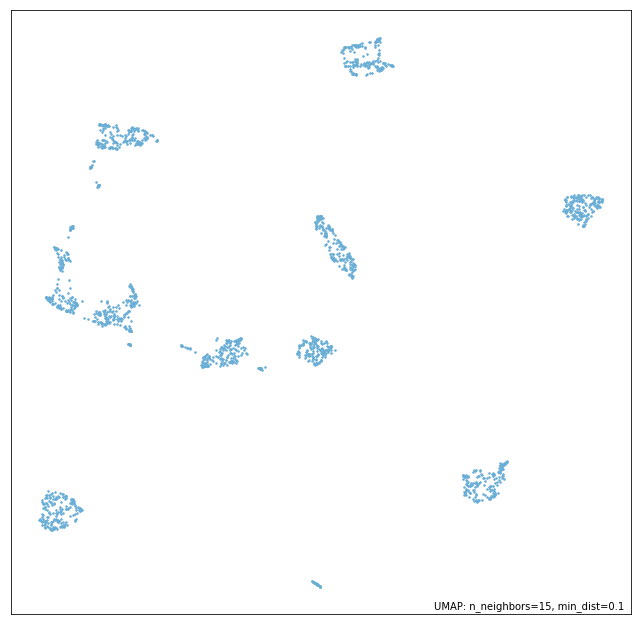As you can see we immediately get a scatterplot of the UMAP embedding. Note that the function automatically selects a point-size based on the data density, and watermarks the image with the UMAP parameters that were used (this will include the metric if it is non-standard). The function also returns the matplotlib axes object associated to the plot, so further matplotlib functions, such as adding titles, axis labels etc. can be applied by the user if required.

It is common for data passed to UMAP to have an associated set of labels, which may have been derived from ground-truth, from clustering, or via other means. In such cases it is desirable to be able to color the scatterplot according to the labelling. We can do this by simply passing the array of label information in with the `labels` keyword. The `umap.plot.points` function will color the data with a categorical colormap according to the labels provided.

```umap.plot.points(mapper, labels=pendigits.target)
```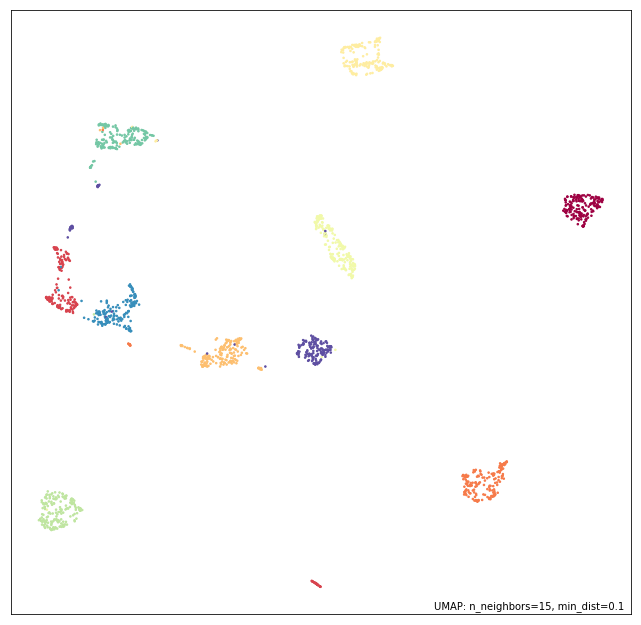Alternatively you may have extra data that is continuous rather than categorical. In this case you will want to use a continuous colormap to shade the data. Again this is straightforward to do – pass in the continuous data with the `values` keyword and data will be colored accordingly using a continuous colormap.

Furthermore, if you don’t like the default color choices the `umap.plot.points` function offers a number of ‘themes’ that provide predefined color choices. Themes include:

• fire

• viridis

• inferno

• blue

• red

• green

• darkblue

• darkred

• darkgreen

Here we will make use of the ‘fire’ theme to demonstrate how simple it is to change the aesthetics.

```umap.plot.points(mapper, values=pendigits.data.mean(axis=1), theme='fire')
```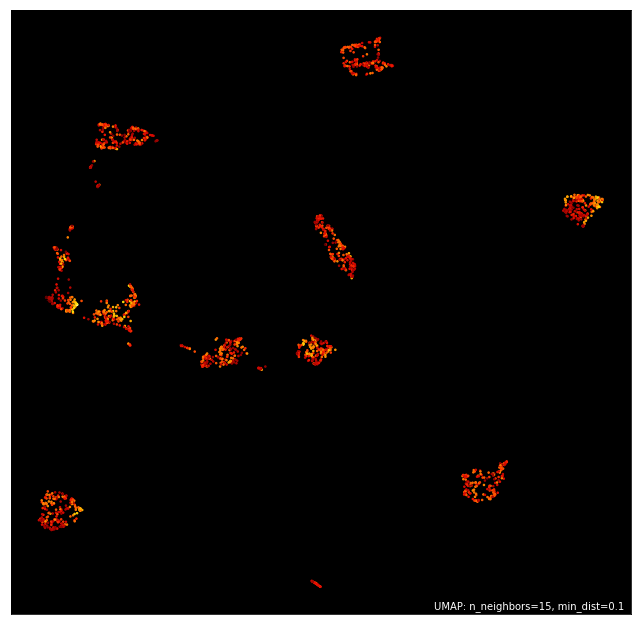If you want greater control you can specify exact colormaps and background colors. For example here we want to color the data by label, but use a black background and use the ‘Paired’ colormap for the categorical coloring (passed as `color_key_cmap`; the `cmap` keyword defines the continuous colormap).

```umap.plot.points(mapper, labels=pendigits.target, color_key_cmap='Paired', background='black')
```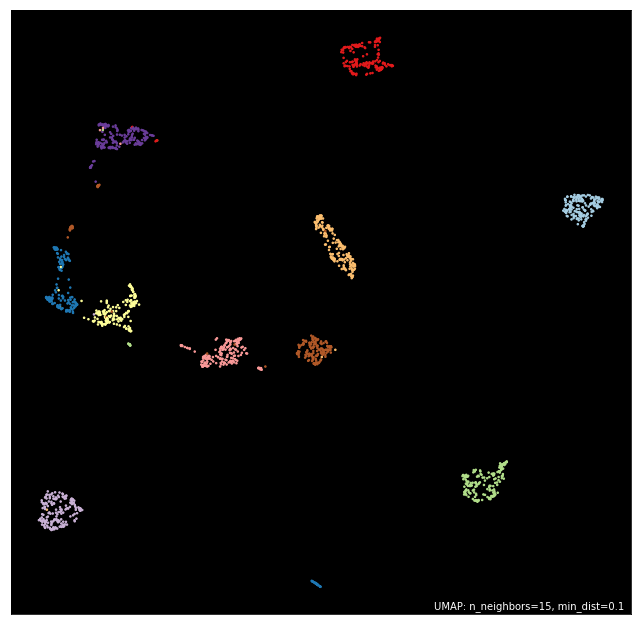Many more options are available including a `color_key` to specify a dictionary mapping of discrete labels to colors, `cmap` to specify the continous colormap, or the width and height of the resulting plot. Again, this does not provide comprehensive control of the plot aesthetics, but the goal here is to provide a simple to use interface rather than the ability for the user to fine tune all aspects – users seeking such control are far better served making use of the individual underlying packages (matplotlib, datashader, and bokeh) by themselves.

## Plotting larger datasets¶

Once you have a lot of data it becomes easier for a simple scatter plot to lie to you. Most notably overplotting, where markers for points overlap and pile up on top of each other, can deceive you into thinking that extremely dense clumps may only contain a few points. While there are things that can be done to help remedy this, such as reducing the point size, or adding an alpha channel, few are sufficient to be sure the plot isn’t subtly lying to you in some way. This essay in the datashader documentation does an excellent job of describing the issues with overplotting, why the obvious solutions are not quite sufficient, and how to get around the problem. To make life easier for users the `umap.plot` package will automatically switch to using datashader for rendering once your dataset gets large enough. This helps to ensure you don’t get fooled by overplotting. We can see this in action by working with one of the larger datasets such as Fashion-MNIST.

```mapper = umap.UMAP().fit(fmnist.data)
```

Having fit the data with UMAP we can call `umap.plot.points` exactly as before, but this time, since the data is large enough to have potential overplotting, datashader will be used in the background for rendering.

```umap.plot.points(mapper)
```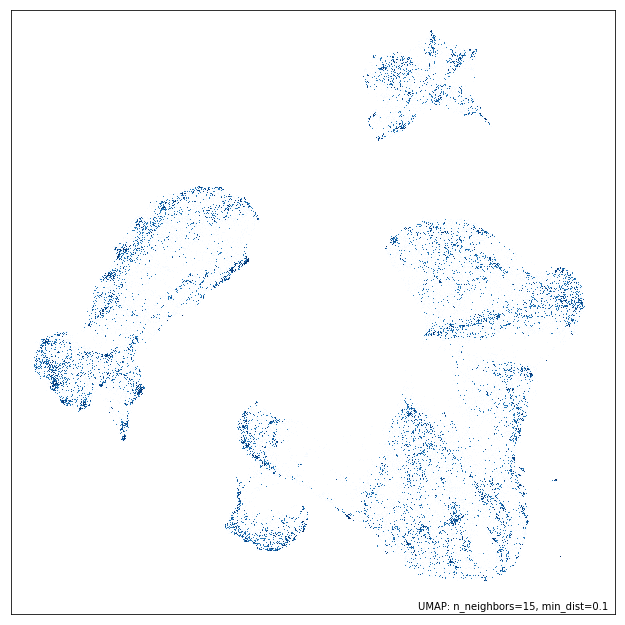All the same plot options as before hold, so we can color by labels, and apply the same themes, and it will all seamlessly use datashader for the actual rendering. Thus, regardless of how much data you have `umap.plot.points` will render it well with a transparent user interface. You, as a user, don’t need to worry about switching to plotting with datashader, or how to convert your plotting to its slightly different API – you can just use the same API and trust the results you get.

```umap.plot.points(mapper, labels=fmnist.target, theme='fire')
```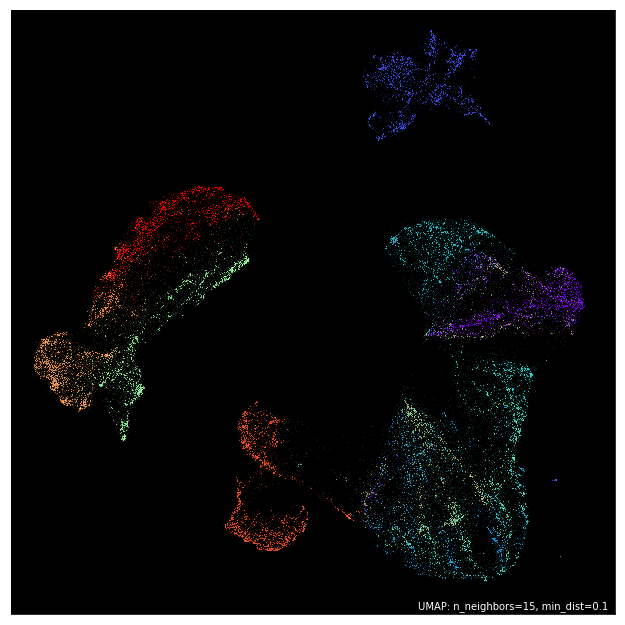## Interactive plotting, and hover tools¶

Rendering good looking static plots is important, but what if you want to be able to interact with your data – pan around, and zoom in on the clusters to see the finer structure? What if you want to annotate your data with more complex labels than merely colors? Wouldn’t it be good to be able to hover over data points and get more information about the individual point? Since this is a very common use case `umap.plot` tries to make it easy to quickly generate such plots, and provide basic utilities to allow you to have annotated hover tools working quickly. Again, the goal is not to provide a comprehensive solution that can do everything, but rather a simple to use and consistent API to get users up and running fast.

To make a good example of this let’s use a subset of the Fashion MNIST dataset. We can quickly train a new mapper object on that.

```mapper = umap.UMAP().fit(fmnist.data[:30000])
```

The goal is to be able to hover over different points and see data associated with the given point (or points) under the cursor. For this simple demonstration we’ll just use the target information of the point. To create hover information you need to construct a dataframe of all the data you would like to appear in the hover. Each row should correspond to a source of data points (appearing in the same order), and the columns can provide whatever extra data you would like to display in the hover tooltip. In this case we’ll need a dataframe that can include the index of the point, its target number, and the actual name of the type of fashion item that target corresponds to. This is easy to quickly put together using pandas.

```hover_data = pd.DataFrame({'index':np.arange(30000),
'label':fmnist.target[:30000]})
hover_data['item'] = hover_data.label.map(
{
'0':'T-shirt/top',
'1':'Trouser',
'2':'Pullover',
'3':'Dress',
'4':'Coat',
'5':'Sandal',
'6':'Shirt',
'7':'Sneaker',
'8':'Bag',
'9':'Ankle Boot',
}
)
```

For interactive use the `umap.plot` package makes use of bokeh. Bokeh has several output methods, but in the approach we’ll be outputting inline in a notebook. We have to enable this using the `output_notebook` function. Alteratively we could use `output_file` or other similar options – see the bokeh documentation for more details.

```umap.plot.output_notebook()
```
Now we can make an interactive plot using `umap.plot.interactive`. This has a very similar API to the `umap.plot.points` approach, but also supports a `hover_data` keyword which, if passed a suitable dataframe, will provide hover tooltips in the interactive plot. Since bokeh allows different outputs, to display it in the notebook we will have to take the extra stop of calling `show` on the result.
```p = umap.plot.interactive(mapper, labels=fmnist.target[:30000], hover_data=hover_data, point_size=2)